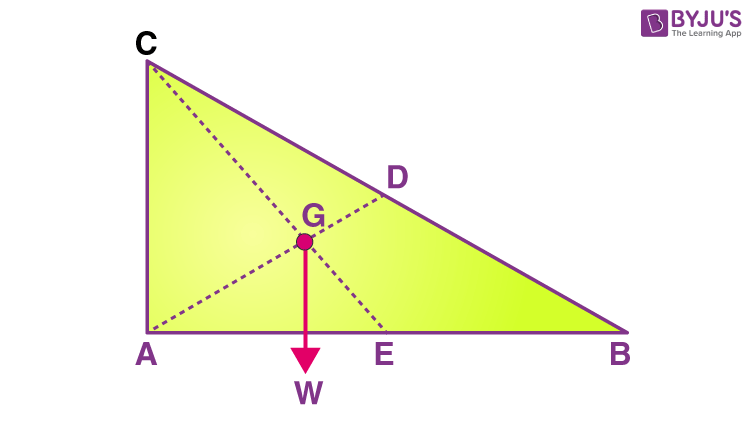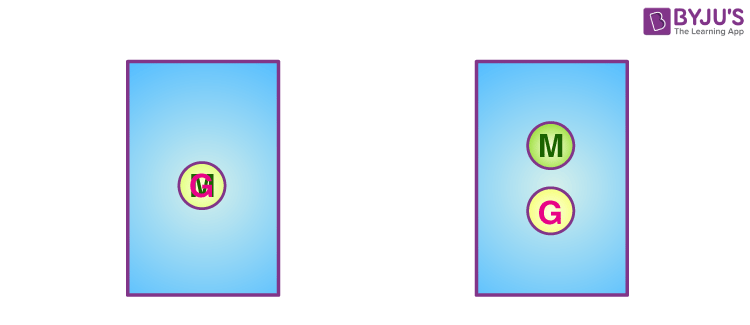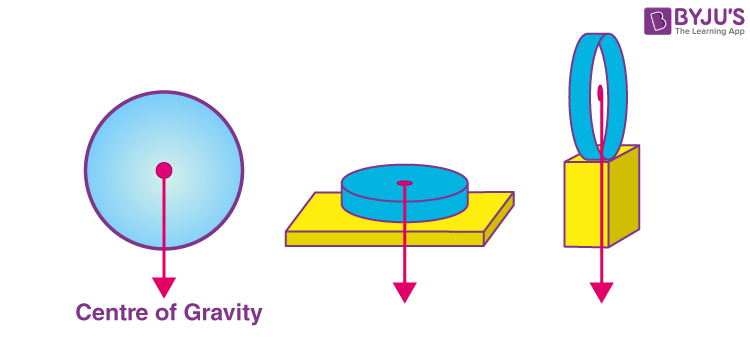# Centre of Gravity

One way to look at gravity is to look at it as a force that pulls things downward more precisely towards the centre of the Earth. But it doesn’t always work like that! Sometimes gravity causes things to topple and turn over, especially if they are high up and unbalanced. No one understands this better than tightrope walkers. While tiptoeing on the high wire, they often wobble from side to side to entertain us, yet they hardly ever fall. Instinctively they understand the physics of forces and manage to stay firmly on the rope. If you, like them, understand a simple concept known as the centre of gravity, you consider balancing a child’s play!

## What is the Centre of Gravity?

The Centre of gravity is a theoretical point in the body where the body’s total weight is thought to be concentrated. It is important to know the centre of gravity because it predicts the behaviour of a moving body when acted on by gravity. It is also useful in designing static structures such as buildings and bridges.

In a uniform gravitational field, the centre of gravity is identical to the centre of mass. Yet, the two points do not always coincide. For the Moon, the centre of mass is very close to its geometric centre. However, its centre of gravity is slightly towards the Earth due to the stronger gravitational force on the Moon’s near side.

In a symmetrically shaped object formed of homogenous material, the centre of gravity may match the body’s geometric centre. However, an asymmetrical object composed of various materials with different masses is likely to have its centre of gravity located at some distance away from its geometric centre. In hollow bodies or irregularly shaped bodies, the centre of gravity lies at a point external to the physical material.

## Calculating the Centre of Gravity

In the next few sections, we will learn how to determine the centre of gravity of objects. In general, determining the centre of gravity may be complicated because the mass and weight may not be uniformly distributed throughout the object.

### Determining the Centre of Gravity for Symmetrical Bodies

For simple shaped object, the centre of gravity can be determined by the following ways:

1. When we balance an object with the help of a string or an edge, the point at which the object balances is the centre of gravity.
2. Another simple physical procedure employed to determine the centre of gravity is as follows:

Suspending the plate given in the figure by attaching a cord to point A and then by attaching the cord at point C, the centre of gravity of the plate can be located.The line AD is vertical when the plate is suspended from A; likewise, the line CE is vertical when suspended from C. The centre of gravity of the plate lies at the intersection of AD and CE. When an object is suspended from any single point, its centre of gravity lies directly beneath that point.

### Determining the Centre of Gravity for Asymmetrical Bodies

We make use of calculus to determine the centre of gravity when the mass is not distributed uniformly. We denote the integration of a continuous function with respect to weight using the symbol S dw. Then, the centre of gravity is determined as follows:

$$\begin{array}{l}cg\times W=SxdW\end{array}$$

Here, x is the distance from a reference line, dw is an increment of weight, and W is the total weight of the object.

We know that

$$\begin{array}{l}W = mg\end{array}$$

But, the mass of an object is equal to

$$\begin{array}{l}m=\rho V\end{array}$$

Combining both the equations, we get

$$\begin{array}{l}W=g\rho V\end{array}$$

Solving,

$$\begin{array}{l}dW=g\rho dV\end{array}$$
$$\begin{array}{l}dW=g*\rho(x,y,z)* dxdydz\end{array}$$

If we have a functional form of the mass distribution, we can solve the equation for the centre of gravity.

$$\begin{array}{l}cg * W = g * SSS x * \rho (x,y,z) dx dy dz\end{array}$$

SSS in the equation indicates a triple integral over dx. dy. and dz.

## Difference Between Centre of Mass and Centre of Gravity

It is common to assume the centre of mass and the centre of gravity to be the same, but this is not the case.

The centre of mass is a point at which mass distribution is equal in all directions, and it doesn’t depend upon the gravitational field. The centre of gravity is a point in an object where the distribution of weight is equal in all directions, and it does depend on the gravitational field.

However, an object’s centre of mass and centre of gravity lies at the same point in a uniform gravitational field.In the above figure, the object on the left is in a uniform gravitational field and has an overlapping centre of gravity and mass. For the object on the right, the gravitational field is stronger towards its base, and the centre of gravity is below the centre of mass.

#### How can a ring be balanced if its centre of gravity lies outside?

The centre of gravity of a uniform circular ring does not lie in its centre but at its geometric centre. Although it lies outside the mass, the centre of gravity of a circular ring balances itself when placed on another object, as shown in the figure. The gravitational force acts through the supporting object.## Frequently Asked Questions on Centre of Gravity

Q1

### Who discovered the centre of gravity?

Archimedes of Syracuse discovered the centre of gravity.

Q2

### How does the Centre of gravity affect the balance?

The centre of gravity affects the stability of objects. The lower the centre of gravity (G) is, the more stable the object. The higher it is, the more likely the object is to topple over if it is pushed. Racing cars have shallow centres of gravity so that they can corner rapidly without turning over.

Q3

### Why is the centre of gravity important?

The Centre of gravity vastly simplifies calculations involving gravitation and dynamics to treat the mass of an object as if it is concentrated at one point.

Q4

### Where is the centre of gravity in a human body?

In an anatomical position, the centre of gravity lies approximately anterior to the second sacral vertebra. However, since human beings do not remain fixed in the anatomical position, the precise location of the COG changes constantly with every new position of the body and limbs.

Q5

### How does centre of gravity affect stability

The position of the centre of gravity of an object affects its stability. The lower the centre of gravity (G) is, the more stable the object. The higher it is, the more likely the object is to topple over if it is pushed.

Stay tuned to BYJU’S and Fall in Love with Learning!

Test your knowledge on Centre Of Gravity